# 单智能体强化学习算法

## Behavior Regularized Offline Reinforcement Learning

Posted by MY on September 15, 2020

## 一、问题

Offline RL中常用的两类方法为：

• 使用target Q-value的ensemble来减少预估误差以稳定Q的学习
• 考虑到未见过的state-action对更可能产生过估计的Q值，因此将学习到的策略向行为策略进行规范和约束 但是现有方法中的这些设计对于Offline RL的学习效果的影响是未知的，本文着眼于上述第二种情况，设计了一个框架behavior regularized actor critic (BRAC)，将现有的一些约束学习策略的工作如BCQ、BEAR等涵盖在内，以对现有方法的不同组件的重要性进行分析。

## 二、解法

### 2.1 约束学习策略

• value penalty (vp)：在值函数中加入惩罚项
• policy regularization (pr)：在策略中加入正则项

value penalty的方式如下。定义penalized value function：

$V_{D}^{\pi}(s)=\sum_{t=0}^{\infty} \gamma^{t} \mathbb{E}_{s_{t} \sim P_{t}^{\pi}(s)}\left[R^{\pi}\left(s_{t}\right)-\alpha D\left(\pi\left(\cdot \mid s_{t}\right), \pi_{b}\left(\cdot \mid s_{t}\right)\right)\right]$

$\min _{Q_{\psi}} \mathbb{E}_{\left(s, a, r, s^{\prime}\right) \sim \mathcal{D}}\left[\left(r+\gamma\left(\bar{Q}\left(s^{\prime}, a^{\prime}\right)-\alpha \hat{D}\left(\pi_{\theta}\left(\cdot \mid s^{\prime}\right), \pi_{b}\left(\cdot \mid s^{\prime}\right)\right)\right)-Q_{\psi}(s, a)\right)^{2}\right]$

$\max _{\pi_{\theta}} \mathbb{E}_{\left(s, a, r, s^{\prime}\right) \sim \mathcal{D}}\left[\mathbb{E}_{a^{\prime \prime} \sim \pi_{\theta}(\cdot \mid s)}\left[Q_{\psi}\left(s, a^{\prime \prime}\right)\right]-\alpha \hat{D}\left(\pi_{\theta}(\cdot \mid s), \pi_{b}(\cdot \mid s)\right)\right]$

### 2.2 散度函数$D$的选择与散度函数采样估计$\hat{D}$的实现

CV中常用的方法是使用image的patches作为正负样本。考虑到RL的特性，使用这种需要设计如何获取pathces的较为复杂的方式会为RL的训练带来更大的难度，因此，CURL使用instance区分而不是patch区分。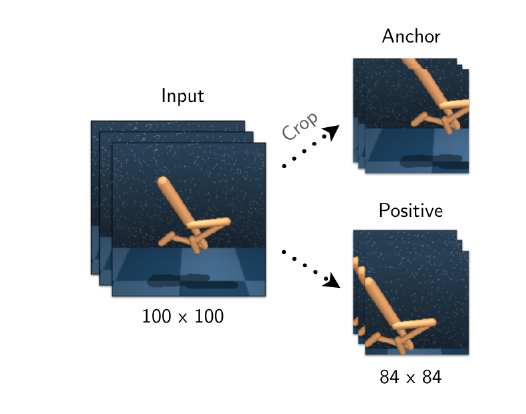#### 2.2.1 Kernel MMD

BEAR中使用的便是Kernel MMD：

$\operatorname{MMD}_{k}^{2}\left(\pi(\cdot \mid s), \pi_{b}(\cdot \mid s)\right)=\underset{x, x^{\prime} \sim \pi(\cdot \mid s)}{\mathbb{E}}\left[K\left(x, x^{\prime}\right)\right]-2 \mathbb{E}_{x \sim \pi(\cdot \mid s)}[K(x, y)]+\underset{y \sim \pi_{b}(\cdot \mid s)}{\mathbb{E}}\left[\mathbb{E}_{y, y^{\prime} \sim \pi_{b}(\cdot \mid s)}\left[K\left(y, y^{\prime}\right)\right]\right.$

$\hat{\pi}_{b}:=\underset{\hat{\pi}}{\operatorname{argmax}} \mathbb{E}_{\left(s, a, r, s^{\prime}\right) \sim \mathcal{D}}[\log \hat{\pi}(a \mid s)]$

#### 2.2.2 KL Divergence

$D_{\mathrm{KL}}\left(\pi_{\theta}(\cdot \mid s), \pi_{b}(\cdot \mid s)\right)=\mathbb{E}_{a \sim \pi_{\theta}(\cdot \mid s)}\left[\log \pi_{\theta}(a \mid s)-\log \pi_{b}(a \mid s)\right]$

$D_{f}(p, q)=\mathbb{E}_{x \sim p}[f(q(x) / p(x))]=\max _{g: \mathcal{X}_{\mapsto} \operatorname{dom}\left(f^{*}\right)} \mathbb{E}_{x \sim q}[g(x)]-\mathbb{E}_{x \sim p}\left[f^{*}(g(x))\right]$

#### 2.2.3 Wasserstein Distance

$W(p, q)=\sup _{g:\|g\|_{L} \leq 1} \mathbb{E}_{x \sim p}[g(x)]-\mathbb{E}_{x \sim q}[g(x)]$

#### 2.2.4 BEAR

BEAR方法使用了基于采样的Kernel MMD和min-max形式的Q值ensemble。除此之外，此外，BEAR自适应地将正则化权重$\alpha$训练为Lagriagian乘数：它将Kernel MMD距离设置为$\epsilon>0$的阈值，如果当前平均散度高于阈值，则增大$\alpha$；如果低于阈值，则减小$\alpha$。

#### 2.2.5 BCQ

BCQ没有使用正则项，即vp和pr里的$\alpha$都为0.

## 三、实验内容

BEAR中动态学习正则化权重的方式没有固定权重好：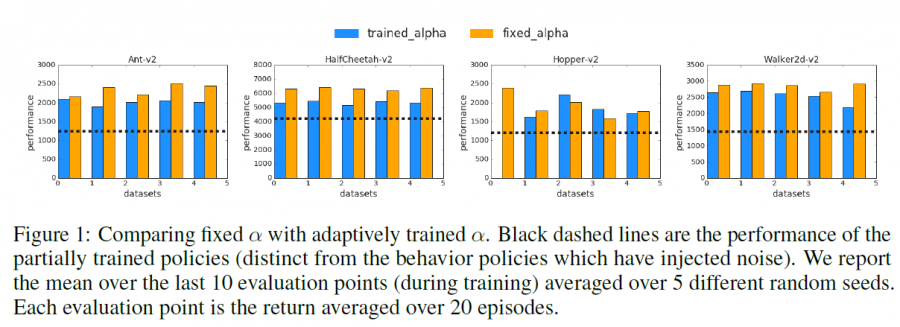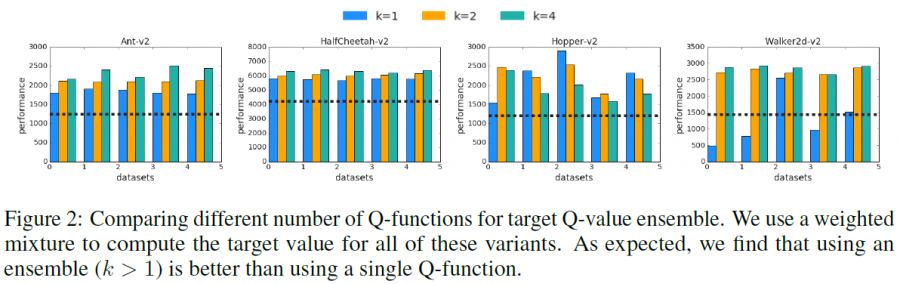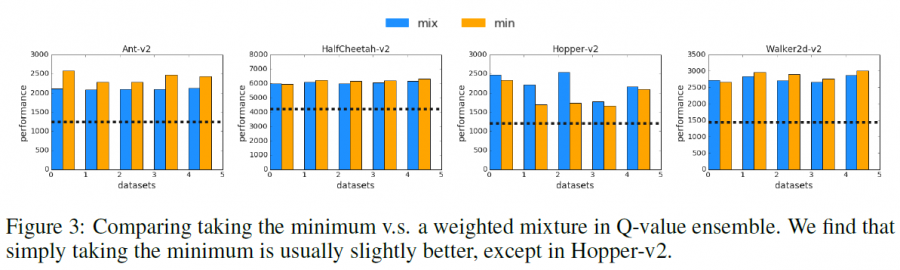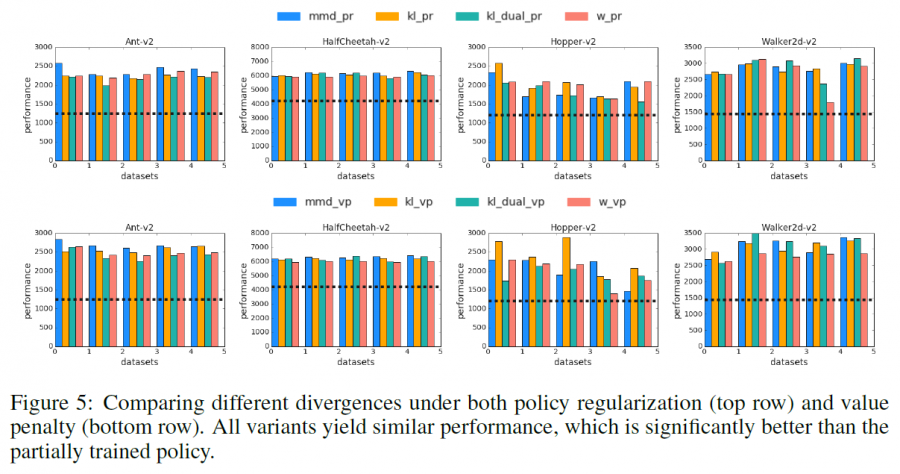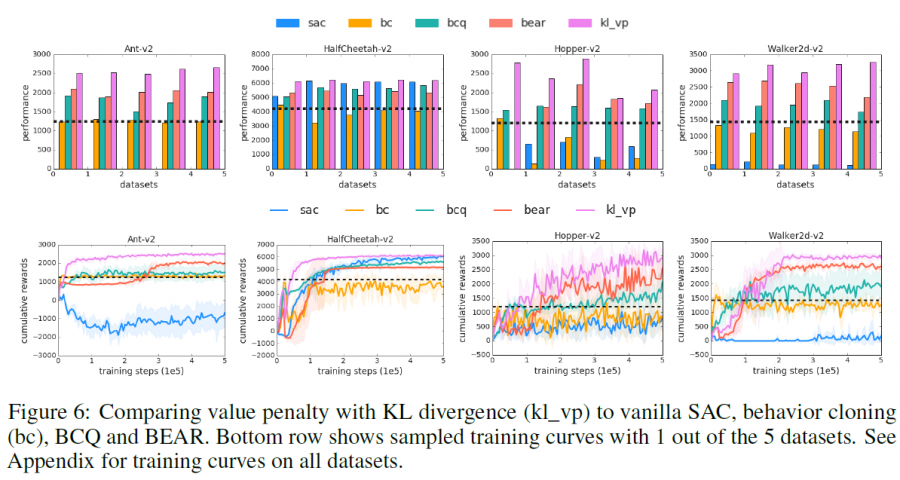## 四、缺点

• 提出的各种正则化方法，结果非常接近，这在某种程度上限制了本文作为RL研究的实用准则（虽然反向说明了使用不同的正则化方式区别不大）
• value penalty与policy regularization两种方法区别很小，且不是作者原创的贡献

## 五、优点

• 基本上为约束行为策略这一类的方法构造了一个完备的算法框架
• 实验充分对比了不同组件的重要程度，说明了Q-ensemble和动态调整正则化权重的作用则不是特别大

##### Share